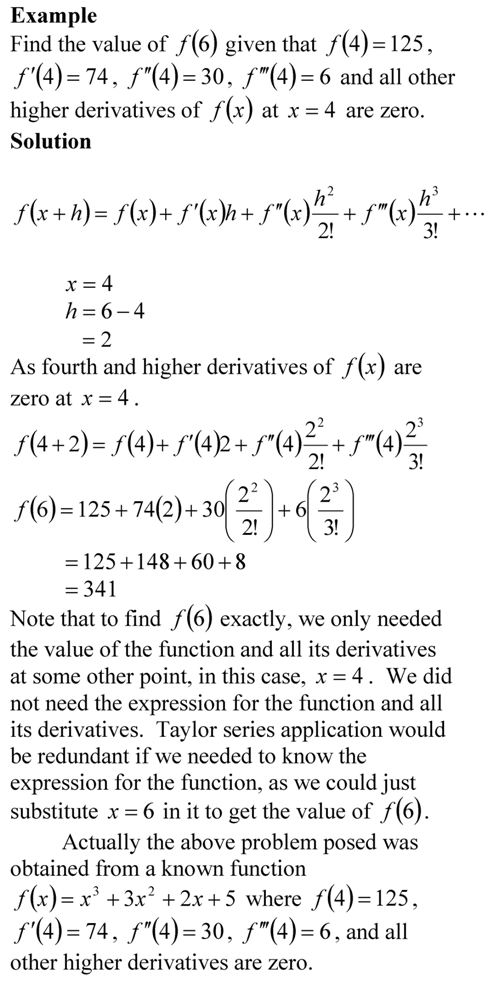# Taylor series example

If Archimedes were to quote Taylor’s theorem, he would have said, “Give me the value of the function  and the value of all (first, second, and so on) its derivatives at a single point, and I can give you the value of the function at any other point”.

It is very important to note that the Taylor’s theorem is not asking for the expression of the function and its derivatives, just the value of the function and its derivatives at a single point.

Now the fine print: Yes, all the derivatives have to exist and be continuous between x and x+h, the point where you are wanting to calculate the function at. However, if you want to calculate the function approximately by using the nth order Taylor polynomial, then 1st, 2nd,…., nth derivatives need to exist and be continuous in the closed interval [x,x+h], while the (n+1)th derivative needs to exist and be continuous in the open interval (x,x+h).## 0 thoughts on “Taylor series example”

1.Sandeep Mishra says:

its was a very good example but only one will not satisfy the student…..sorry but in a way what i wanna say is that i want you guys to post some more examples so that we students can get an idea about how the theorem works….thank you

2.Sandeep Mishra says:

its was a very good example but only one will not satisfy the student…..sorry but in a way what i wanna say is that i want you guys to post some more examples so that we students can get an idea about how the theorem works….thank you

3.bushra says:

plz help me to solve the example! use taylor’s theorem with n=2 to approximate (1+x)3,x>-1

4.bushra says:

plz help me to solve the example! use taylor’s theorem with n=2 to approximate (1+x)3,x>-1